Intended for healthcare professionals
Home/5. Differences between means: type I and type II errors and power

# 5. Differences between means: type I and type II errors and power

We saw in Chapter 3 that the mean of a sample has a standard error, and a mean that departs by more than twice its standard error from the population mean would be expected by chance only in about 5% of samples. Likewise, the difference between the means of two samples has a standard error. We do not usually know the population mean, so we may suppose that the mean of one of our samples estimates it. The sample mean may happen to be identical with the population mean but it more probably lies somewhere above or below the population mean, and there is a 95% chance that it is within 1.96 standard errors of it.
Consider now the mean of the second sample. If the sample comes from the same population its mean will also have a 95% chance of lying within 196 standard errors of the population mean but if we do not know the population mean we have only the means of our samples to guide us. Therefore, if we want to know whether they are likely to have come from the same population, we ask whether they lie within a certain range, represented by their standard errors, of each other.

## Large sample standard error of difference between means

If SD1 represents the standard deviation of sample 1 and SD2 the standard deviation of sample 2, n1 the number in sample 1 and n2 the number in sample 2, the formula denoting the standard error of the difference between two means is:(5.1)
The computation is straightforward.
Square the standard deviation of sample 1 and divide by the number of observations in the sample: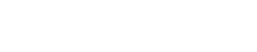(1)
Square the standard deviation of sample 2 and divide by the number of observations in the sample: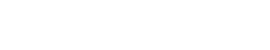(2)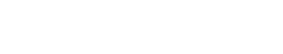Take the square root, to give equation 5.1. This is the standard error of the difference between the two means.

## Large sample confidence interval for the difference in two means

From the data in the general practitioner wants to compare the mean of the printers’ blood pressures with the mean of the farmers’ blood pressures. The figures are set out first as in table 5.1 (which repeats table 3.1 ).
Table 5.1Analysing these figures in accordance with the formula given above, we have:
The difference between the means is 88 – 79 = 9 mmHg.
For large samples we can calculate a 95% confidence interval for the difference in means as9 – 1.96 x 0.81 to 9 + 1.96 x 0.81 which is 7.41 to 10.59 mmHg.
For a small sample we need to modify this procedure, as described in Chapter 7.

## Null hypothesis and type I error

In comparing the mean blood pressures of the printers and the farmers we are testing the hypothesis that the two samples came from the same population of blood pressures. The hypothesis that there is no difference between the population from which the printers’ blood pressures were drawn and the population from which the farmers’ blood pressures were drawn is called the null hypothesis.
But what do we mean by “no difference”? Chance alone will almost certainly ensure that there is some difference between the sample means, for they are most unlikely to be identical. Consequently we set limits within which we shall regard the samples as not having any significant difference. If we set the limits at twice the standard error of the difference, and regard a mean outside this range as coming from another population, we shall on average be wrong about one time in 20 if the null hypothesis is in fact true. If we do obtain a mean difference bigger than two standard errors we are faced with two choices: either an unusual event has happened, or the null hypothesis is incorrect. Imagine tossing a coin five times and getting the same face each time. This has nearly the same probability (6.3%) as obtaining a mean difference bigger than two standard errors when the null hypothesis is true. Do we regard it as a lucky event or suspect a biased coin? If we are unwilling to believe in unlucky events, we reject the null hypothesis, in this case that the coin is a fair one.
To reject the null hypothesis when it is true is to make what is known as a type I error . The level at which a result is declared significant is known as the type I error rate, often denoted by α. We try to show that a null hypothesis is unlikely , not its converse (that it is likely), so a difference which is greater than the limits we have set, and which we therefore regard as “significant”, makes the null hypothesis unlikely . However, a difference within the limits we have set, and which we therefore regard as “non-significant”, does not make the hypothesis likely.
A range of not more than two standard errors is often taken as implying “no difference” but there is nothing to stop investigators choosing a range of three standard errors (or more) if they want to reduce the chances of a type I error.

## Testing for differences of two means

To find out whether the difference in blood pressure of printers and farmers could have arisen by chance the general practitioner erects the null hypothesis that there is no significant difference between them. The question is, how many multiples of its standard error does the difference in means difference represent? Since the difference in means is 9 mmHg and its standard error is 0.81 mmHg, the answer is: 9/0.81 = 11.1. We usually denote the ratio of an estimate to its standard error by “z”, that is, z = 11.1. Reference to Table A (Appendix table A.pdf) shows that z is far beyond the figure of 3.291 standard deviations, representing a probability of 0.001 (or 1 in 1000). The probability of a difference of 11.1 standard errors or more occurring by chance is therefore exceedingly low, and correspondingly the null hypothesis that these two samples came from the same population of observations is exceedingly unlikely. The probability is known as the P value and may be written P < 0.001.
It is worth recapping this procedure, which is at the heart of statistical inference. Suppose that we have samples from two groups of subjects, and we wish to see if they could plausibly come from the same population. The first approach would be to calculate the difference between two statistics (such as the means of the two groups) and calculate the 95% confidence interval. If the two samples were from the same population we would expect the confidence interval to include zero 95% of the time, and so if the confidence interval excludes zero we suspect that they are from a different population. The other approach is to compute the probability of getting the observed value, or one that is more extreme , if the null hypothesis were correct. This is the P value. If this is less than a specified level (usually 5%) then the result is declared significant and the null hypothesis is rejected. These two approaches, the estimation and hypothesis testing approach, are complementary. Imagine if the 95% confidence interval just captured the value zero, what would be the P value? A moment’s thought should convince one that it is 2.5%. This is known as a one sided P value , because it is the probability of getting the observed result or one bigger than it. However, the 95% confidence interval is two sided, because it excludes not only the 2.5% above the upper limit but also the 2.5% below the lower limit. To support the complementarity of the confidence interval approach and the null hypothesis testing approach, most authorities double the one sided P value to obtain a two sided P value (see below for the distinction between one sided and two sided tests).
Sometimes an investigator knows a mean from a very large number of observations and wants to compare the mean of her sample with it. We may not know the standard deviation of the large number of observations or the standard error of their mean but this need not hinder the comparison if we can assume that the standard error of the mean of the large number of observations is near zero or at least very small in relation to the standard error of the mean of the small sample.
This is because in equation 5.1 for calculating the standard error of the difference between the two means, when n1 is very large then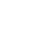becomes so small as to be negligible. The formula thus reduces towhich is the same as that for standard error of the sample mean, namelyConsequently we find the standard error of the mean of the sample and divide it into the difference between the means.
For example, a large number of observations has shown that the mean count of erythrocytes in men is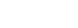In a sample of 100 men a mean count of 5.35 was found with standard deviation 1.1. The standard error of this mean is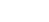,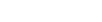. The difference between the two means is 5.5 – 5.35 = 0.15. This difference, divided by the standard error, gives z = 0.15/0.11 = 136. This figure is well below the 5% level of 1.96 and in fact is below the 10% level of 1.645 (see table A ). We therefore conclude that the difference could have arisen by chance.

## Alternative hypothesis and type II error

It is important to realise that when we are comparing two groups a non-significant result does not mean that we have proved the two samples come from the same population – it simply means that we have failed to prove that they do not come from the population. When planning studies it is useful to think of what differences are likely to arise between the two groups, or what would be clinically worthwhile; for example, what do we expect to be the improved benefit from a new treatment in a clinical trial? This leads to a study hypothesis , which is a difference we would like to demonstrate. To contrast the study hypothesis with the null hypothesis, it is often called the alternative hypothesis . If we do not reject the null hypothesis when in fact there is a difference between the groups we make what is known as a type II error . The type II error rate is often denoted as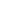. The power of a study is defined as 1 –and is the probability of rejecting the null hypothesis when it is false. The most common reason for type II errors is that the study is too small.
The concept of power is really only relevant when a study is being planned (see Chapter 13 for sample size calculations). After a study has been completed, we wish to make statements not about hypothetical alternative hypotheses but about the data, and the way to do this is with estimates and confidence intervals.(1)

## Common questions

Why is the P value not the probability that the null hypothesis is true?
A moment’s reflection should convince you that the P value could not be the probability that the null hypothesis is true. Suppose we got exactly the same value for the mean in two samples (if the samples were small and the observations coarsely rounded this would not be uncommon; the difference between the means is zero). The probability of getting the observed result (zero) or a result more extreme (a result that is either positive or negative) is unity, that is we can be certain that we must obtain a result which is positive, negative or zero. However, we can never be certain that the null hypothesis is true, especially with small samples, so clearly the statement that the P value is the probability that the null hypothesis is true is in error. We can think of it as a measure of the strength of evidence against the null hypothesis, but since it is critically dependent on the sample size we should not compare P values to argue that a difference found in one group is more “significant” than a difference found in another.

## References

Gardner MJ Altman DG, editors. Statistics with Confidence . London: BMJ Publishing Group. Differences between means: type I and type II errors and power

## Exercises

5.1 In one group of 62 patients with iron deficiency anaemia the haemoglobin level was 1 2.2 g/dl, standard deviation 1.8 g/dl; in another group of 35 patients it was 10.9 g/dl, standard deviation 2.1 g/dl.
What is the standard error of the difference between the two means, and what is the significance of the difference? What is the difference? Give an approximate 95% confidence interval for the difference. 5.2 If the mean haemoglobin level in the general population is taken as 14.4 g/dl, what is the standard error of the difference between the mean of the first sample and the population mean and what is the significance of this difference?

## UK Jobs

Penn Hill Surgery: Salaried GP - Penn Hill Surgery